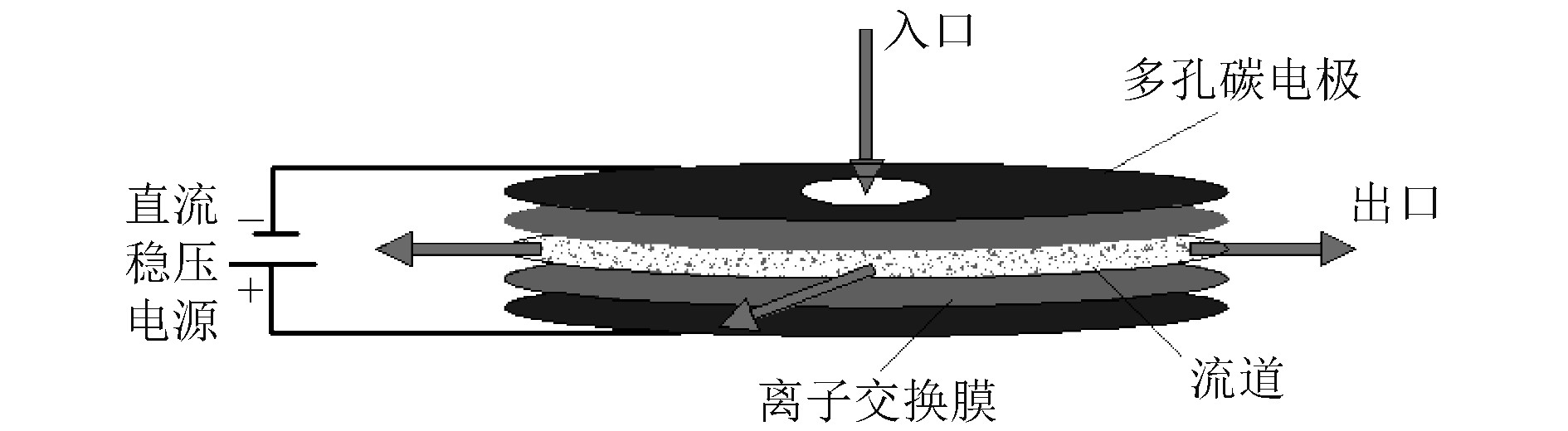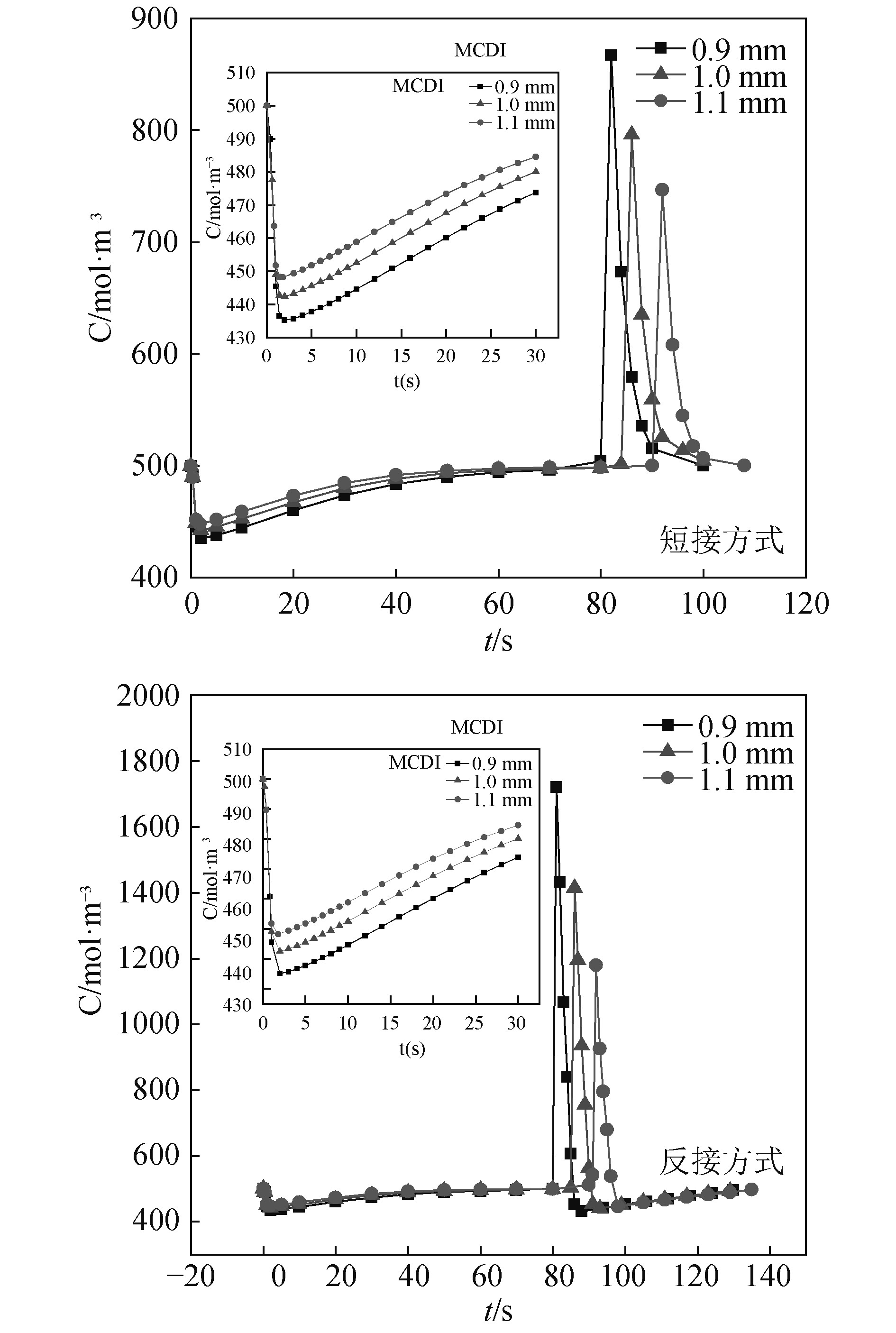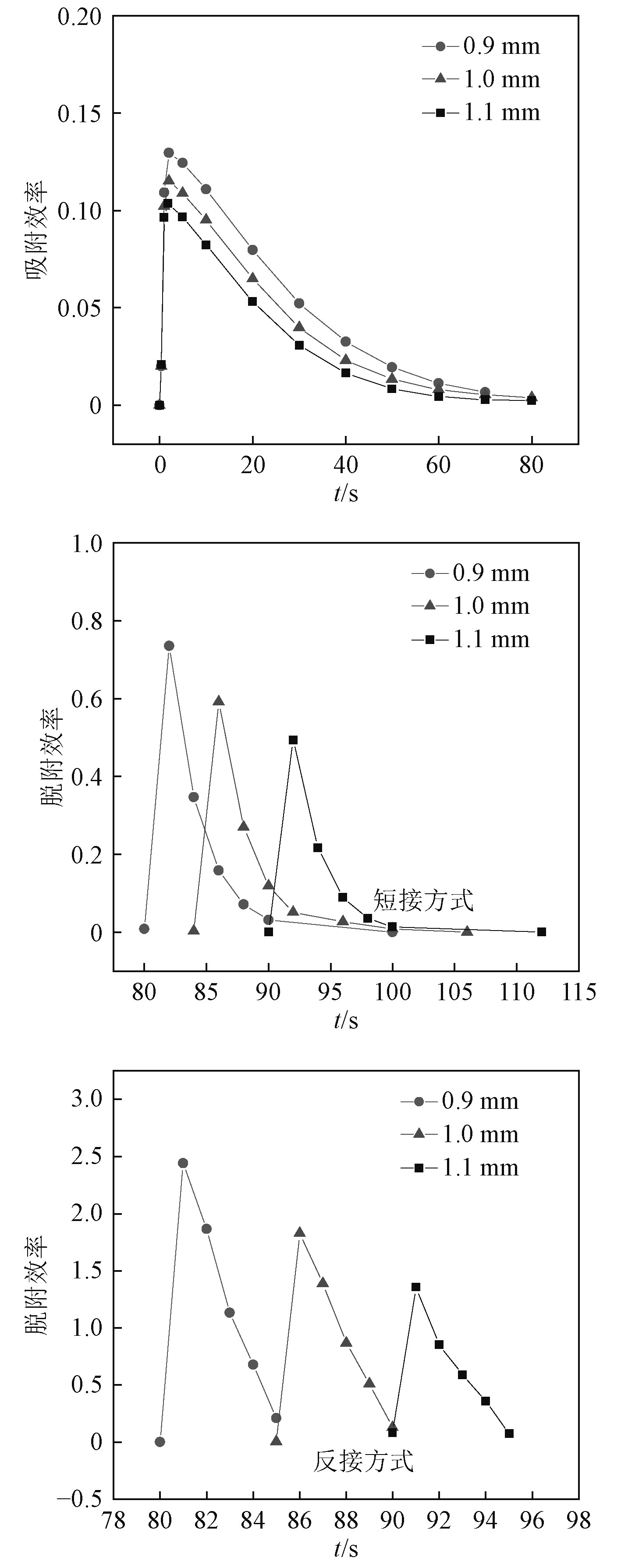﻿ 膜电容去离子法海水淡化装置单元脱盐过程的数值模拟
 舰船科学技术2020, Vol. 42Issue (6): 110-114    DOI: 10.3404/j.issn.1672-7649.2020.06.022PDF

Numerical simulation of desalting process in seawater desalination plant by membrane capacitive deionization
XIAO Min, JIN Miao-miao, YAO Shou-guang
School of Energy and Power Engineering, Jiangsu University of Science and Technology, Zhenjiang 212003, China
Abstract: Based on the middle inflow type desalting module in membrane capacitance method, a three-dimensional transient analysis model of desalting unit was established. Then the applicability of the model was verified by linear regression fitting of simulation results, and the desalting unit was analyzed by numerical simulation. The results show that with the increase of inlet aperture, the minimum outlet concentration of MCDI desalting unit increases, while the maximum decreases. The larger the inlet aperture, the longer the desalting unit reaches the adsorption saturation time, and the smaller the adsorption efficiency, yet the more the desorption time increases, and the higher the desorption efficiency peak. Under the same inlet aperture, the maximum outlet concentration in reverse desorption mode both achieves higher than that in short.
Key words: membrane capacitive deionization     middle inflow type     numerical simulation
0 引　言

1 模型建立 1.1 MCDI模型几何结构图 1 中部流入式MCDI模型几何结构图 Fig. 1 Geometric structure diagram of MCDI model in the middle inflow type
1.2 数学模型

 $\frac{{\partial {c_{sp}}\left( y \right)}}{{\partial t}} = - \frac{{{J_{ion}}\left( y \right)}}{{{\delta _{sp}}}} - {v_{sp}}\frac{{\partial {{\text{c}}_{sp}}\left( y \right)}}{{\partial y}}\text{。}$ (1)

2个膜/溶液的界面上都具有Donnan平衡，且两者分别分布在膜边缘与流道的接触面和膜边缘与电极的接触面。这2个Donnan势如下式：

 ${\Delta {\rm{\phi }}}_{{\rm{d}}{\rm{o}}{\rm{n}}{\rm{n}}{\rm{a}}{\rm{n}}}={\rm{sin}}{{{h}}}^{-1}\frac{\omega {{X}}}{2{{c}}_{{\rm{s}}{\rm{a}}{\rm{l}}{\rm{t}}}}\text{。}$ (2)

 ${{\text{J}}_{{\text{ion}},{\text{mem}}}}\left( y \right) = - \frac{{{D_{mem}}}}{{{\delta _{mem}}}}\left( {{c_{{\text{T}},{\text{mem}}}}\left( y \right) - \omega X\Delta {\phi _{{\text{mem}}}}\left( y \right)} \right)\text{。}$ (3)

 $\frac{{\partial {c_{sp,i}}}}{{\partial t}} = - \frac{{{J_{ion,i}}}}{{{\delta _{sp}}}} - \frac{M}{{{\tau _{sp}}}}\left( {{c_{sp,i}} - {c_{sp,i - 1}}} \right)\text{。}$ (4)

 $\begin{split} & \frac{1}{2}{V_{{\text{cell}}}}/{V_{\text{T}}} = \Delta {\phi _{{\text{sp}},{\text{half}}}} + \Delta {\phi _{{\text{donnan}},{\text{membrane}}/{\text{spacer}}}} + \\ & \Delta {\phi _{{\text{mem}}}} - \Delta {\phi _{{\text{donnan}},{\text{membrane}}/{\text{electrode}}}} + \Delta {\phi _{{\text{elec}}}} + \\ & {{{\left( {\Delta {\phi _{\text{d}}} + \Delta {\phi _{{\text{St}}}}} \right)}_{{\text{micropores}}}}} \;{\text{。}} \end{split}$ (5)

1.3 边界条件及模型参数 1.3.1 入口与出口边界条件

MCDI单元模型包含流场、离子浓度场、电场等，因此该模型边界条件如下：

 $\left\{ {\begin{array}{*{20}{c}} {{{V = }}{{{v}}_{{\rm{in}}}}}\text{，}\\ {{{p = }}{{{p}}_{{\rm{out}}}}}\text{，}\\ {\nabla {{P}} \cdot \overrightarrow {{n}} {\rm{ = 0}}}\text{。} \end{array}} \right.$ (6)

 $\overrightarrow {{u}} {\rm{ = }}{{{v}}_{{{in}}}}{\rm{, }}{{{c}}_{{i}}}{{ = c}}_{{i}}^{{\rm{ref}}}{{,T = }}{{{T}}_{{{in}}}}\text{。}$ (7)

 $\overrightarrow {{u}} {\rm{ = 0, }}{{{c}}_{{i}}}{{ = c}}_{{i}}^0\text{。}$ (8)
1.3.2 模型参数表 1 模型参数 Tab.1 Model parameters
2 模拟结果与分析 2.1 边界条件及模型参数表 2 模型验证拟合结果 Tab.2 Model fitting results

Lagergren准一级和准二级动力学方程为：

 $\ln\left(1-\frac{{q}_{t}}{{q}_{e}}\right)=-{K}_{1}t\text{，}$ (9)
 $\frac{1}{{{{q}}}_{{{t}}}}=\frac{1}{{K}_{2}{q}_{e}^{2}}\left(\frac{1}{{{t}}}\right)+\frac{1}{{{{q}}}_{{{e}}}}\text{。}$ (10)

2.2 入口孔径对脱盐性能的影响 2.2.1 两种脱附方式下的结果分析图 2 MCDI脱盐单元在不同入口孔径下2种脱附方式的脱盐曲线图 Fig. 2 Desalting curves of MCDI desalting module under different inlet aperture in two desorption methods

2.2.2 吸、脱附效率图 3 MCDI脱盐单元在不同入口孔径下2种脱附方式的吸、脱附效率图 Fig. 3 Adsorption and desorption efficiency charts of MCDI desalting module under different inlet aperture in two desorption methods

3 结　语

1）对模拟得到中部流入式MCDI脱盐模拟过程中Na+出口浓度的数值结果进行线性回归拟合研究表明，本文所建立的中部流入式膜电容法脱盐分析模型适用于对MCDI脱盐单元的数值模拟。

2）MCDI脱盐单元的出口最低浓度随入口孔径的增大而增大，且入口孔径越大，其达到吸附饱和的时间越长，但脱盐单元的吸附效率越小；随着入口孔径的增大，出口最高浓度逐渐递减，脱附时间相应增加，脱盐单元能够达到的脱附效率峰值也越大，同一入口孔径条件下，反接脱附方式下能够达到的最高出口浓度均高于短接脱附方式。

  BIENER J, STADERMANN M, SUSS M, et al. Advanced carbon aerogels for energy applications[J]. Energy and Environmental Science, 2011, 4(03): 656-667. DOI:10.1039/c0ee00627k  SIMON P, GOGOTSI Y. Materials for electrochemical capacitors[J]. Nature Materials, 2008, 7(11): 845-854. DOI:10.1038/nmat2297  SHPIGEL N, LEVI M D, SIGALOV S, et al. In situ hydrodynamic spectroscopy for structure characterization of porous energy storage electrodes[J]. Nature Materials, 2016, 15(05): 570-575. DOI:10.1038/nmat4577  GUYES E N, SHOCRON A N, SIMANOVSKI A, et al. A one-dimensional model for water desalination by flow-through electrode capacitive deionization[J]. Desalination, 2017, 415: 8-13. DOI:10.1016/j.desal.2017.03.013  F HE, BIESHEUVEL P M, BAZANT M Z, et al. Theory of water treatment by capacitive deionization with redox active porous electrodes[J]. Water Research, 2018, 132: 282-291. DOI:10.1016/j.watres.2017.12.073  JIMOH O, JERINA Z, ABDUL H, et al. A comprehensive review on recently developed carbon based nanocomposites for capacitive deionization: From theory to practice[J]. Separation and Purification Technology, 2018, 207: 291-320. DOI:10.1016/j.seppur.2018.06.046  赵飞, 苑志华, 钟鹭斌, 等. 电容去离子技术及其电极材料研究进展[J]. 水处理技术, 2016, 42(05): 38-44.  BIESHEUVEL P M, ZHAO R, PORADA S, et al. Theory of membrane capacitive deionization including the effect of the electrode pore space[J]. Journal of Colloid and Interface Science, 2011, 360(01): 239-248. DOI:10.1016/j.jcis.2011.04.049  HASSANVAND A, CHEN G Q, WEBLEY P A, et al. A comparison of multicomponent electrosorption in capacitive deionization and membrane capacitive deionization[J]. Water Research, 2018, 131: 100-109. DOI:10.1016/j.watres.2017.12.015  WANG L, LIN S H. Theoretical framework for designing a desalination plant based on membrane capacitive deionization[J]. Water Research, 2019, 158: 359-369. DOI:10.1016/j.watres.2019.03.076  PORADA S, WEINSTEIN L, DASH R, et al. Water desalination using capacitive deionization with microporous carbon electrodes[J]. ACS Applied Materials and Interfaces, 2012, 4(03): 1194-1199. DOI:10.1021/am201683j  ZHAO R, BIESHEUVEL P M, MIEDEMA H, et al. Charge efficiency: A functional tool to probe the double-layer structure inside of porous electrodes and application in the modeling of capacitive deionization[J]. Journal of Physical Chemistry Letters, 2010, 1(01): 205-210. DOI:10.1021/jz900154h  BIESHEUVEL P M. Two-fluid model for the simultaneous flow of colloids and fluids in porous media[J]. Journal of Colloid and Interface Science, 2011, 355(02): 389-395. DOI:10.1016/j.jcis.2010.12.006  BIESHEUVEL P M, VANDERWAL A. Membrane capacitive deionization[J]. Journal of Membrane Science, 2010, 346(02): 256-262. DOI:10.1016/j.memsci.2009.09.043  LI H B, PAN L K, ZHANG Y, et al. Kinetics and thermodynamics study for electrosorption of NaCl onto carbon nanotubes and carbon nanofibres electrodes[J]. Chemical Physics Letters, 2010, 485(1-3): 161-166. DOI:10.1016/j.cplett.2009.12.031